Lesson 4.3: Conversions and Calculator

Access Lesson 4.3 slides here

Contents:

• Unit conversions
• Data exploration
• Calculator
• Easter eggs

We live in a world where we often have to convert from one unit measure to another. You want to convert from degrees Celsius to degrees Fahrenheit, or number of tablespoons to number of cups. Google has this fantastic ability to do automatic conversions combined with the ability to do calculations. You can use Google to do calculations very easily.

Here is the general pattern for doing unit conversions: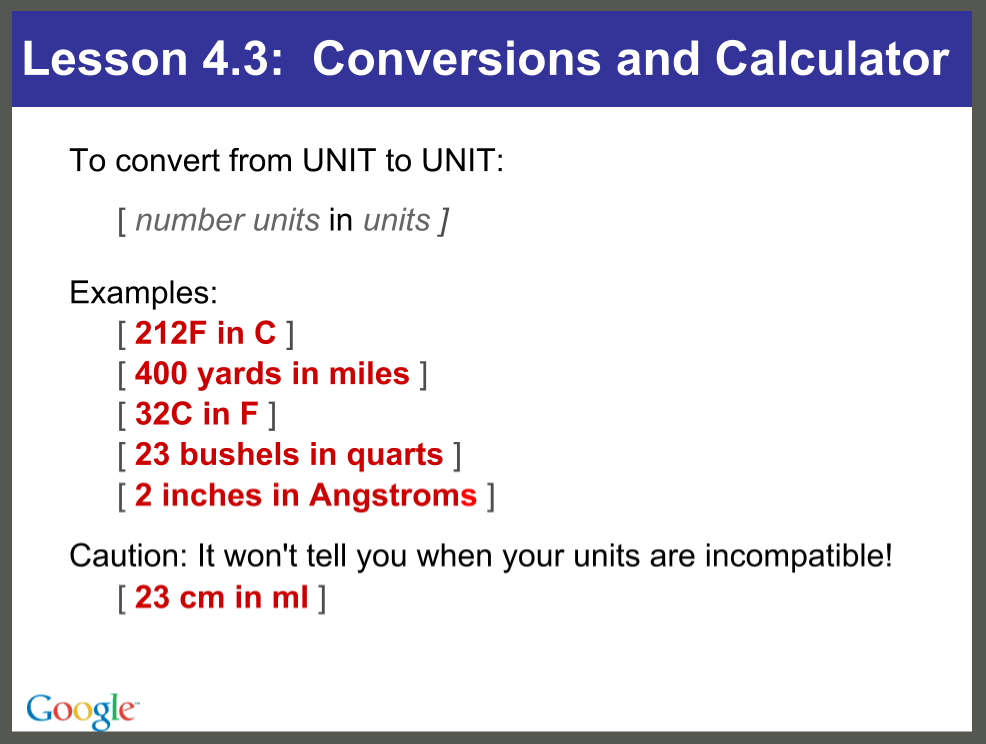Figure: Examples of conversions from the class slides.

To convert from units to units, use the pattern [number units in units]. For example, I can go to Google and type the boiling point of water [212f in c] and get the conversion from Fahrenheit to Celsius.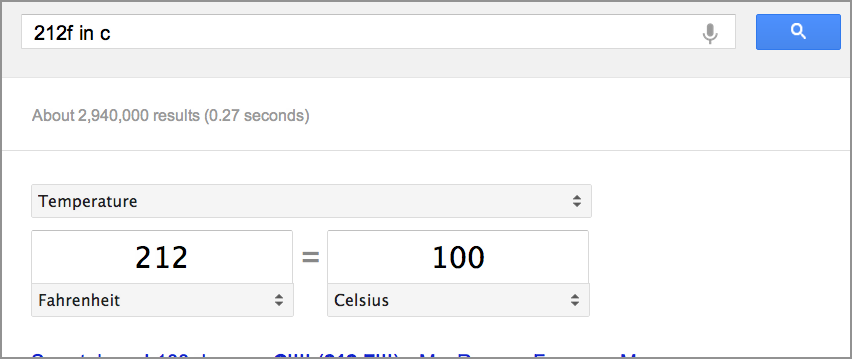Figure: Conversion result for query [212f in c].

In this case, the boiling point of water in Fahrenheit is exactly 100 degrees Celsius. That's pretty straightforward.

Or, try another one: [400 yards in miles].Figure: Conversion result for query [400 yards in miles].

Use a number, like 400; use the unit measure name, like yards, inches, centimeters, whatever; the word "in"; and then the other unit—that is, what you want it to be converted into.

If you travel to a part of the word with another measurement system—and you end up buying a cookbook—now you can do the conversions from one unit measure to another.

You can convert units you know to units you don't really understand. Angstroms are a measure of very, very small units. They are usually used for microscopes or electron microscopy. You measure things in Angstroms when you're trying to talk about silicon chips. So [one inch in angstrom] is a quarter billion—two hundred and fifty four million Angstroms is one inch.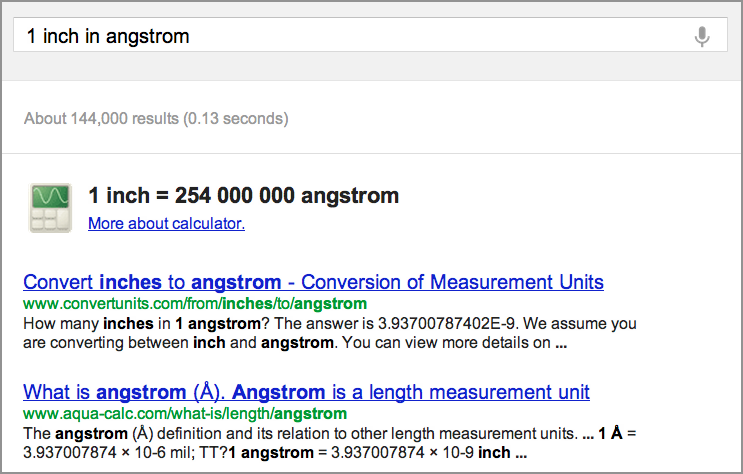Figure: Conversion result for query [one inch in angstrom].

Say you swim 500 yards, and want to see what that is in miles. You can do it immediately.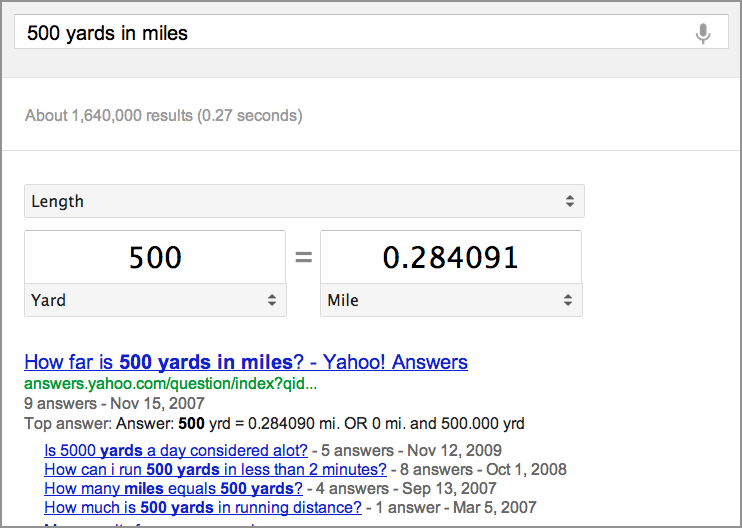Figure: Conversion result for query [500 yards in miles].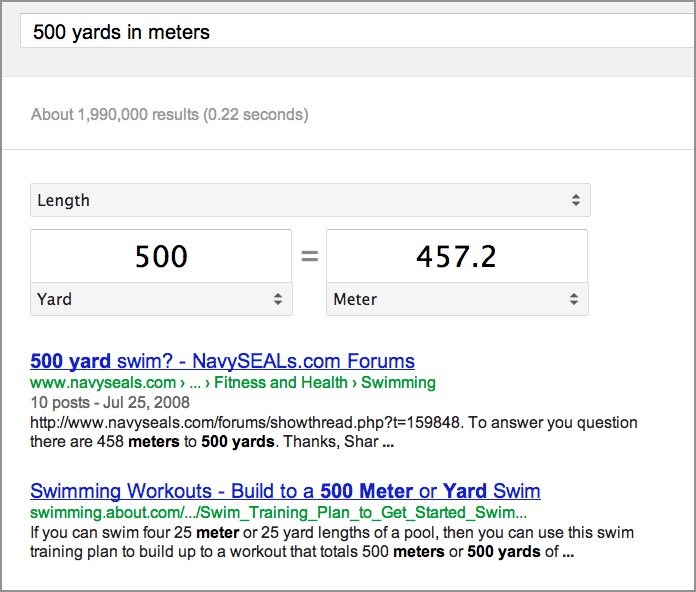Figure: Conversion result for query [500 yards in meters].

Conversions can also be useful for things other than purely scientific and mathematical things.

You may have heard of the book 20,000 Leagues Under the Sea. To what does that title refer? Let’s try [20000 leagues in miles].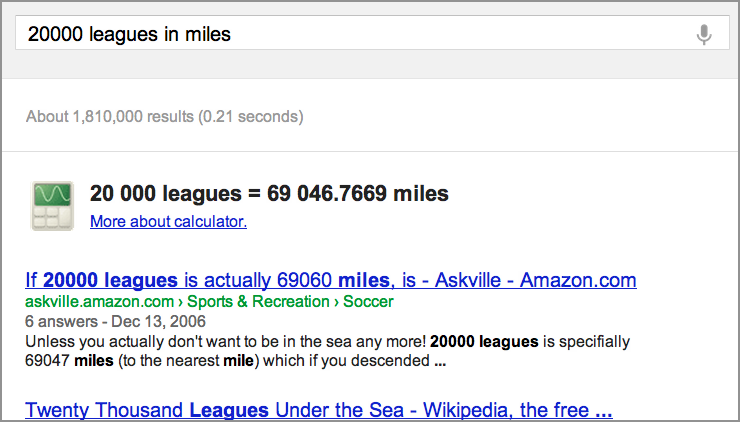Figure: Conversion result for query [20000 leagues in miles].

You can find that 20,000 leagues is 69,000 miles. That's a long way. That is not the depth to which the submarine goes in that book, as many people believe, it is actually the distance that it travels in the course of the novel.

Monetary conversions are also possible. Say you want to know how much is [280 Euros in US dollars] and get the financial conversions.

Please note that there are the normal disclaimers about how you shouldn’t use this for automatic updates for monetary transactions. But it gives you a pretty close to real time sense of what the conversion rate is. To get just a simple conversion rate, type [one euro in usd] and we can see that at the time of writing it is 1.11 US dollars.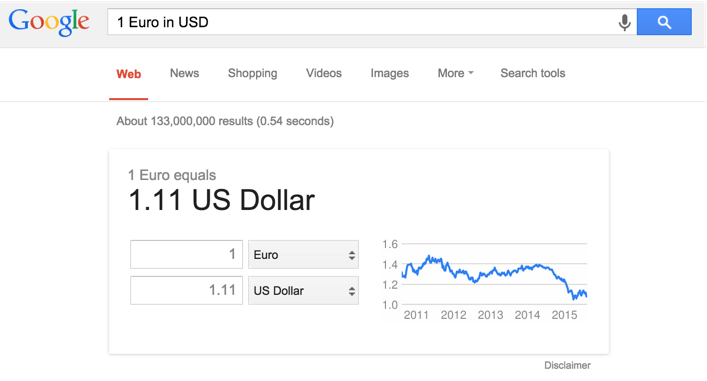Figure: Conversion result for [one euro in usd].

You can convert other kinds of measurements, as well. Perhaps you are increasing a chocolate chip cookie recipe that requires five tablespoons of sugar. But suppose you want to find how many cups you will need if you are multiplying the recipe by four. So you want [20 tablespoons in cups].Figure: Conversion result for query [20 tablespoons in cups].

Rather than counting out 20 tablespoons, you now know you can just get one and a quarter cups of sugar.

As you saw in the last lesson, [population of japan] is one of those questions you can ask very easily.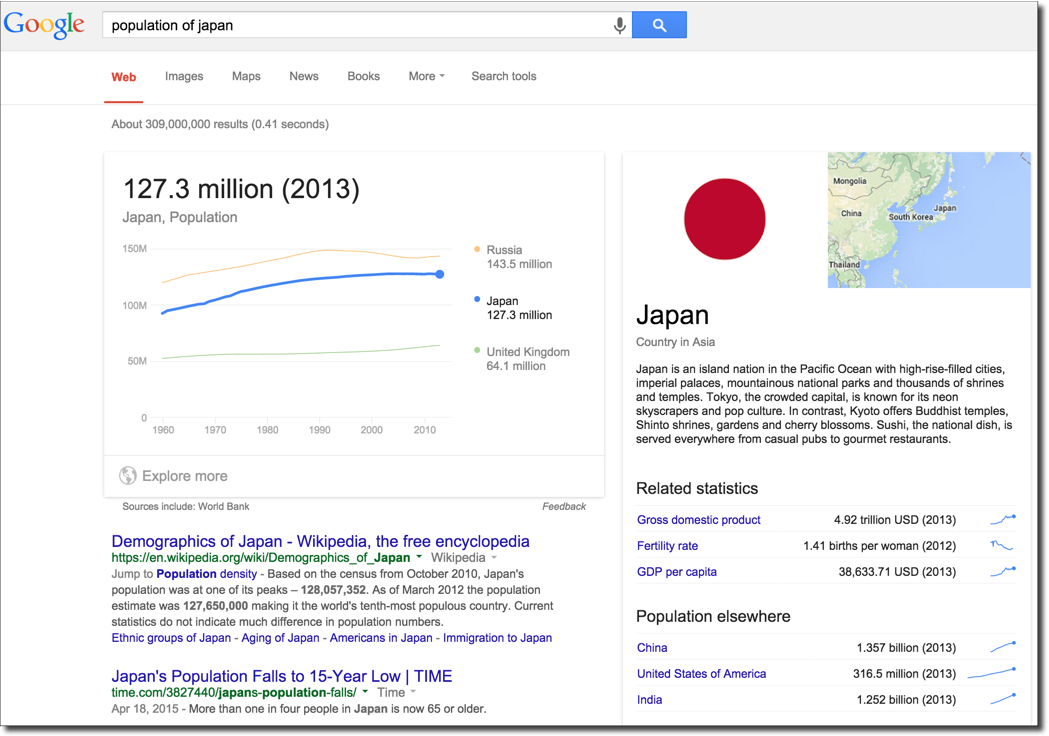Figure: Special features result for [population of japan].

You can even click on the graph in the result, and end up on a data exploration page.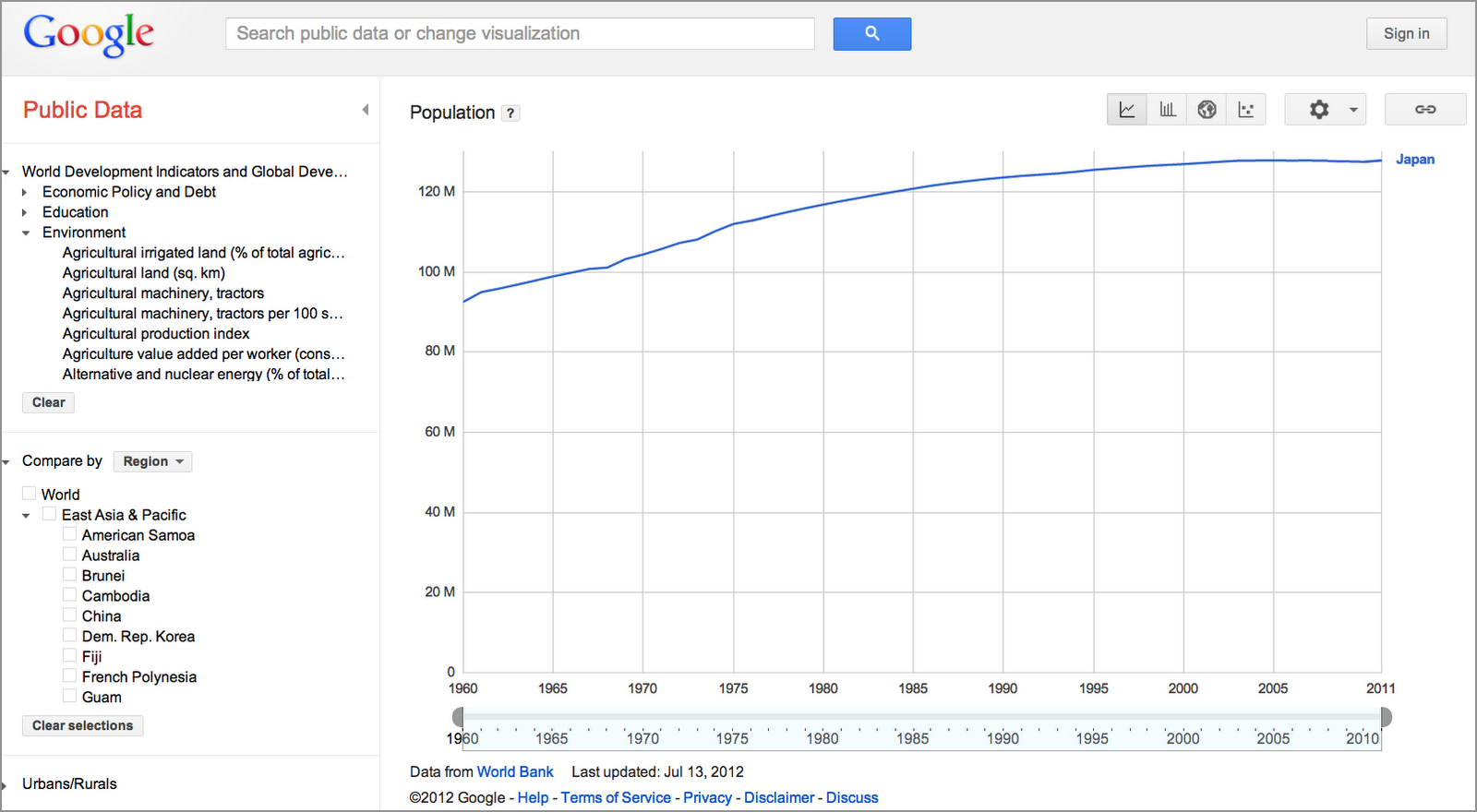Figure: Graph of the changes in the population of Japan, 1900-2011.

You started out looking at just the single number, and now you can look at things like the change in Japan’s population over time since the 1960s.

Another task you can do is to answer simple calculator questions like [one plus two].Figure: Calculator result for query [one plus two].

You can very quickly do calculations like 23 divided by 45 times 121. There's a lot of functionality built in here: try [12% of 124].Figure: Calculator result for the query [12% of 124].

The built-in Google calculator has a a lot of capabilities, including some fun things. Consider this joke we call an Easter egg: [the number of horns on a unicorn] is unsurprisingly one. But what's really fun about this Easter egg is that I can now start to add additional expressive values like [the loneliest number]  and I discover that [the number of horns on a unicorn plus the loneliest number] is two. Who knew?Figure: An “Easter egg” result for the query at [the number of horns on a unicorn plus the loneliest number.

In any event, there's a lot of capability here. Google calculator has a wide range of things it can do.

Test out some of your skills in the next exercise.